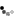Bayesian Inference of High-Dimensional Dynamical Models: The Survival of the Fittest

Speaker: Pierre F.J. Lermusiaux
[Announcement (PDF)]

Speaker Affiliation: Associate Professor,
Department of Mechanical Engineering
Ocean Science and Engineering
Massachusetts Institute of Technology

Date: Thursday, November 3, 2016 at 12 p.m in 37-212
CCE Seminar

We develop and illustrate a dynamics-based Bayesian inference methodology that assimilates sparse observations for the joint inference of the state, parameters, boundary conditions, and initial conditions of dynamical models, but also of the parameterizations, geometry, and model equations themselves. The joint Bayesian inference combines stochastic Dynamically Orthogonal (DO) partial differential equations for reduced-dimension probabilistic prediction with Gaussian Mixture Models for nonlinear filtering and smoothing. The Bayesian model inference is completed by parallelized and analytical computation of marginal likelihoods for multiple candidate models. The classes of models considered correspond to competing scientific hypotheses and differ in complexity and in the representation of specific processes. Within each model class, model equations have unknown parameters and uncertain parameterizations, all of which are estimated. For each model class, the result is a Bayesian update of the joint distribution of the state, parameters, and parameterizations. The combined scientific result is a rigorous Bayesian inference of the marginal distribution of dynamical model equations. Examples are provided for time-dependent fluid and ocean flows. They include the inference of multiscale bottom gravity current dynamics, motivated in part by classic dense water overflows and their relevance to climate monitoring. The Bayesian inference of biogeochemical reaction equations is then presented, illustrating how PDE-based machine learning could rigorously guide the selection and discovery of complex ecosystem or other reaction models. This is joint work with our MSEAS group at MIT.